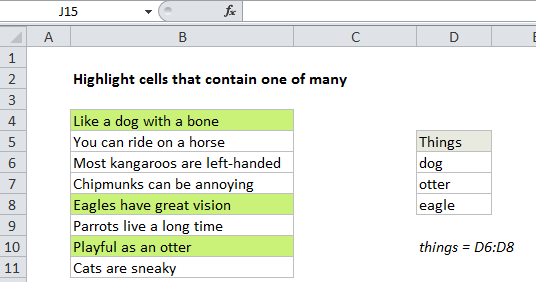## Excel Office

Excel How Tos, Tutorials, Tips & Tricks, Shortcuts

# Highlight cells that contain one of many in Excel

This tutorial shows how to Highlight cells that contain one of many in Excel using the example below;

## Formula

`=SUMPRODUCT(--ISNUMBER(SEARCH(things,A1)))>0`## Explanation

To highlight cells that contain one of many text strings, you can use a formula based on the functions ISNUMBER and SEARCH, together with the SUMPRODUCT function. In the example shown, the conditional formatting applied to B4:B11 is based on this formula:

`=SUMPRODUCT(--ISNUMBER(SEARCH(things,B4)))>0`

### How this formula works

Working from the inside out, this part of the formula searches each cell in B4:B11 for all values in the named range “things”:

`--ISNUMBER(SEARCH(things,B4)`

The SEARCH function returns the position of the value if found, and and the #VALUE error if not found. For B4, the results come back in an array like this:

`{8;#VALUE!;#VALUE!}`

The ISNUMBER function changes all results to TRUE or FALSE:

`{TRUE;FALSE;FALSE}`

The double negative in front of ISNUMBER forces TRUE/FALSE to 1/0:

`{1;0;0}`

The SUMPRODUCT function then adds up the results, which is tested against zero:

`=SUMPRODUCT({1;0;0})>0`

Any non-zero result means at least one value was found, so the formula returns TRUE, triggering the rule.

Worked Example:   How to Check If A Cell Contains Specific Text in Excel

### Ignore empty things

To ignore empty cells in the named range “things”, you can try a modified formula like this:

`=SUMPRODUCT(--ISNUMBER(SEARCH(IF(things<>"",things),B4)))>0`

This works as long as the text values you are testing don’t contain the string “FALSE”. If they do, you can extend the IF function to include a value if false known not to occur in the text (i.e. “zzzz”, “####”, etc.)

Worked Example:   Sum by weekday in Excel

### Case-sensitive option

SEARCH is not case-sensitive. If you need to check case as well, just replace SEARCH with FIND like so:

`=SUMPRODUCT(--ISNUMBER(FIND(things,A1)))>`
Worked Example:   Highlight values greater than in Excel